Main Content

# Resampling Uniformly Sampled Signals

This example shows how to resample a uniformly sampled signal to a new uniform rate. It shows how to reduce the impact of large transients as well as how to remove unwanted high frequency content.

### Rate Conversion by a Rational Factor

The `resample` function performs rate conversion from one sample rate to another. `resample` allows you to upsample by an integral factor, `p`, and subsequently decimate by another integral factor, `q`. In this way you can resample to a rational multiple (`p` / `q`) of the original sample rate.

To use the `resample` function on uniform samples, you must provide both the numerator and denominator of this rational factor. To determine the integers you need, you can use the `rat` function.

Here is an example of how to call `rat` when converting from 48 kHz to 44.1 kHz:

```originalFs = 48000; desiredFs = 44100; [p,q] = rat(desiredFs / originalFs)```
```p = 147 ```
```q = 160 ```

`rat` indicates that you can upsample by 147 and decimate by 160. To verify that this produces the desired rate, multiply `p` / `q` by the original sample rate:

`originalFs * p / q`
```ans = 44100 ```

Once you have the ratio between the new and original sample rates, you can call `resample`.

For example, create a 10 millisecond long 500 Hz sinusoid using the original sample rate of 48 kHz and convert it to 44.1 kHz:

```tEnd = 0.01; Tx = 0:1/originalFs:tEnd; f = 500; x = sin(2*pi*f*Tx); y = resample(x,p,q); Ty = (0:numel(y)-1)/desiredFs; plot(Tx,x,'. ') hold on plot(Ty,y,'o ') hold off legend('Original','Resampled')```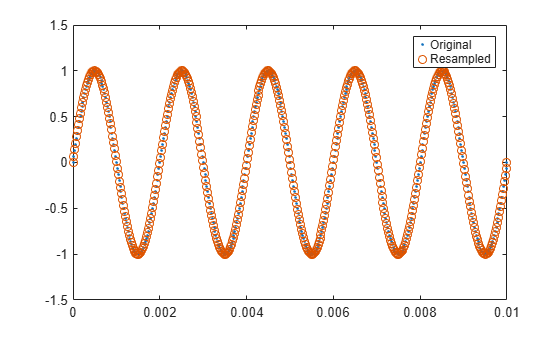For well-behaved signals such as the sinusoid above, simply using `resample` with a carefully chosen `p` and `q` should be enough to reconstruct it properly.

For signals with transients or significant noise, you may wish to have greater control over the poly-phase anti-aliasing filter in `resample`.

### Filtering Transients

The `resample` function uses a filter when it performs rate conversion. This filtering is sensitive to large transients in the signal.

To illustrate this, resample a rectangular pulse:

```x = [zeros(1,120) ones(1,241) zeros(1,120)]; y = resample(x,p,q); plot(Tx,x,'-', Ty,y,'-') legend('Original','Resampled')```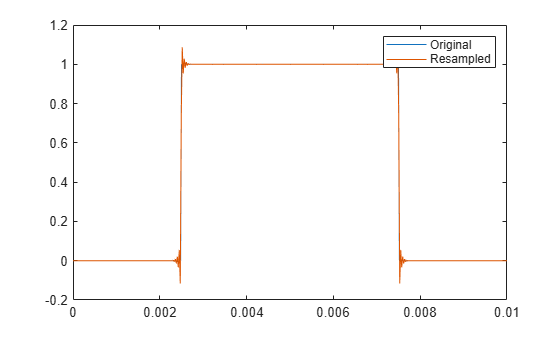The function does a good job of reconstructing the flat regions of the pulse. However, there are spikes at the edges of the pulse.

Zoom in on the edge of the first pulse:

`xlim([2e-3 3e-3])`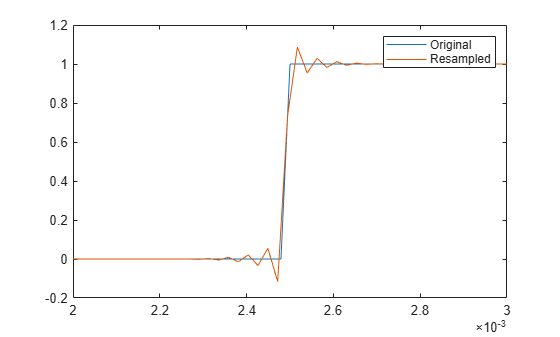There is a damped oscillation in the transition region. You can diminish this oscillation by adjusting the settings of the internal filter.

`resample` allows you to have control over a Kaiser window applied to the anti-aliasing filter that can mitigate some of the edge effects.

Two parameters, `n` and `beta`, control the relative length of the filter and the amount of smoothing it attempts to perform. A larger value of `n` will have a larger filter length. A `beta` of 0 will have no additional smoothing. Larger `beta` values will have larger smoothing. By default, `n` is 10 and `beta` is 5.

A practical way to proceed is to start with the default values and adjust as needed. Here, set `n` to 5 and `beta` to 20.

```n = 5; beta = 20; y = resample(x,p,q,n,beta); plot(Tx,x,'.-') hold on plot(Ty,y,'o-') hold off legend('Original','Resampled') xlim([2e-3 3e-3])```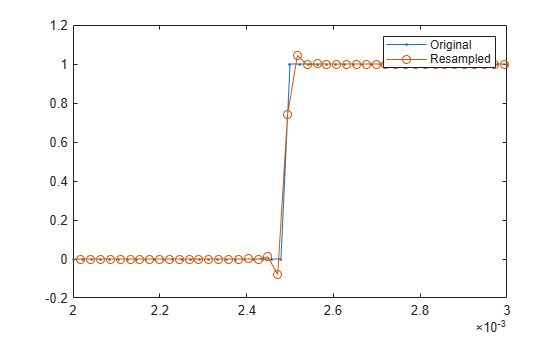The oscillation is significantly reduced.

### Filtering Aliases

The `resample` function is designed to convert sample rates to either higher or lower rates. As a consequence, the frequency cutoff of the anti-aliasing filter is set to the Nyquist frequency of the input or output sample rate (whichever is lower). This default setting allows the `resample` function to cover a wide range of applications.

There are times when direct control of the filter may be beneficial.

To illustrate this, construct and view the spectrogram of a chirp signal sampled at 96 kHz. The chirp signal consists of a sinusoid whose frequency varies quadratically over the entire Nyquist range from 0 Hz to 48 kHz over a duration of eight seconds:

```fs1 = 96000; t1 = 0:1/fs1:8; x = chirp(t1, 0, 8, fs1/2, 'quadratic'); spectrogram(x,kaiser(256,15),220,412,fs1,'yaxis')```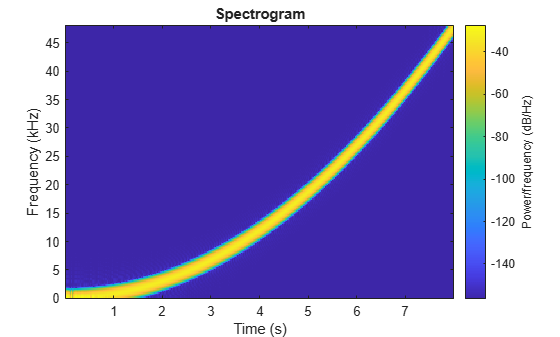Next, convert the chirp to 44.1 kHz using the default settings of `resample` and view the spectrogram:

```fs2 = 44100; [p,q] = rat(fs2/fs1); y = resample(x,p,q); spectrogram(y,kaiser(256,15),220,412,fs2,'yaxis')```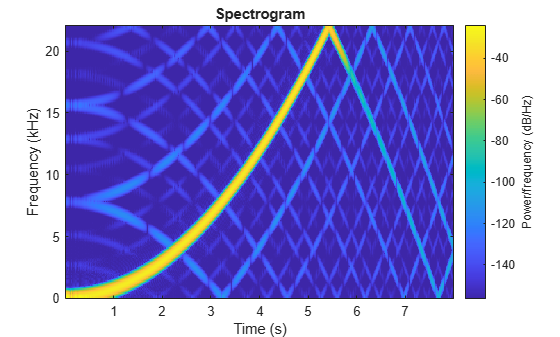Here you can see the original signal as well as unwanted frequency content. Ideally the sinusoid should start at 0 Hz and continue until it reaches the Nyquist frequency of 22.05 kHz at 5.422 s. Instead, there are artifacts introduced due to small discontinuities introduced at the edges of the default filter used for resampling. To prevent these artifacts, you can provide a longer filter with a slightly lower cutoff frequency and greater stop-band rejection than the default filter.

To have proper temporal alignment, the filter should have odd length. The length should be a few times larger than `p` or `q` (whichever is larger). Similarly, divide the desired normalized cutoff frequency by the larger of `p` or `q`. In either case, multiply the resulting coefficients by `p`.

Here is an example of a filter with a cutoff at 98% (0.98) of the output Nyquist frequency with an order of 256 times the decimation factor, windowed with a Kaiser window with a `beta` of 12.

```normFc = .98 / max(p,q); order = 256 * max(p,q); beta = 12; lpFilt = firls(order, [0 normFc normFc 1],[1 1 0 0]); lpFilt = lpFilt .* kaiser(order+1,beta)'; lpFilt = lpFilt / sum(lpFilt); % multiply by p lpFilt = p * lpFilt; % resample and plot the response y = resample(x,p,q,lpFilt); spectrogram(y,kaiser(256,15),220,412,fs2,'yaxis')```Note that the aliases are removed.

### Further Reading

For more information on resampling see the Signal Processing Toolbox.

Reference: fredric j harris, "Multirate Signal Processing for Communications Systems", Prentice Hall, 2004.

## Support

#### Deep Learning for Signal Processing with MATLAB

Download white paper